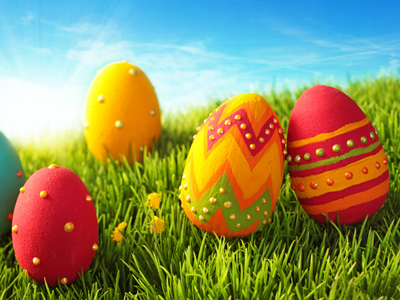You'll find seven easter eggs in this quiz.

# Solving Problems - Money 2 (Medium)

Welcome to the second of our Medium level Eleven plus maths quizzes on Solving Money Problems. In it you will find a mixture of questions – the kind of tests you might well find in real life, if not now, then certainly when you are an adult.

Many of these problems can be solved by using percentages. We use these when we calculate interest or debt so it’s a good thing to get plenty of practice now, long before you have to manage your money as a grown up. You will also be asked to convert one kind of currency to another. Doing this is actually quite easy, as long as you know the exchange rate and whether you have to multiply or divide in order to convert.

Have a go at this quiz and, if you don’t get all the questions right first time, play it again until you do. The more familiar you are with problems like these, the easier you will find your schoolwork and your exams.

1.
A clothes shop is selling last season’s stock with a 60% discount. If a dress now costs £46.80, how much was it before the discount?
£28.08
£44.58
£62.58
£78.00
A 60% discount means that the price has been reduced by 60%. The quickest way to work out what 100% was if £46.80 is 60% is to divide by 6 then times by 10:
46.8 ÷ 6 = 7.8
7.8 x 10 = 78 so the dress originally cost £78
2.
Lucy inherited 25% of her grandfather's business. If the business is worth £1,325,000, what is the value of Lucy’s inheritance?
£993,750
£662,500
£331,250
£325,000
Per cent means per hundred, so 25% = 25100 = 14 and 25% of £1,325,000 means that you have to multiply 25% with £1,325,000: 14 × £1,325,000 = £331,250
3.
Tracey’s bank account pays interest of 2% per year on any money in her account. If Tracey has £894 in her account when the interest is calculated, how much will she earn in interest?
£8.94
£17.88
£26.82
£35.76
Per cent means per hundred, so 2% = 2100 = 150 and 2% of £894 = £894 ÷ 50 = £17.88
4.
Lisa has just arrived in India from the UK, and she wants to change her pounds sterling into Indian Rupees (₹): the exchange rate is £1 = ₹91.6. If Lisa changes £2,500, how many Indian Rupees will she get?
₹22.90
₹2,290
₹22,900
₹229,000
If £1 = ₹91.6, then £2,500 = 2,500 × 91.6 = ₹229,000
5.
It costs a factory £20,000 to build one car. If the factory wants to make a 200% profit on the sale of the car, how much must it sell it for?
£20,000
£40,000
£60,000
£80,000
Profit = Selling Price - Cost Price = £60,000 - £20,000 = £40,000. The factory has made a profit of £40,000. Now £40,000 as a percentage of £20,000 = 40,00020,000 × 100% = 200%. Yes! Don't fall into the trap of thinking that if you sell it at twice its cost that you will make a 200% profit: you have to subtract the original cost from your selling price before you can call it profit
6.
Michael earns a salary of £24,523.50 per annum (per year). He has now been told that he will receive a 10% increase in his salary. How much will Michael earn per annum after his wage rise?
£26,975.85
£25,875.65
£24,775.35
£22,071.15
Per cent means per hundred, so 10% = 10100 = 110 and 10% of £24,523.50 means that you have to multiply £24,523.50 by 1110 (because Michael’s salary has gone up): £24,523.50 x 1110 = £26,975.85. So, Michael will now earn £26,975.85
7.
Stephen has put £10,000 into a savings account. At the end of the year he is paid interest and his balance increases to £10,250. What rate of interest did Stephen’s bank pay?
0.25%
2.5%
25%
250%
Stephen’s balance has gone up by £250 so we have to find what percentage of 10,000 250 is.
Start by turning the problem into a fraction: 25010,000 = 140
Next, turn into a decimal by dividing 1 by 40: 140 = 0.025
Finally, convert the decimal into a percentage by multiplying by 100: 0.025 x 100 = 2.5
Stephen’s bank paid him a 2.5% rate of interest
8.
Elsie bought seven Easter eggs, one for each of her grandchildren. Each Easter egg cost the same amount and Elsie got £4.50 in change from a £50 note. How much would one Easter egg have cost?
£15.50
£45.50
£6.50
£4.50
£50 - £4.50 = £45.50 = what Elsie paid for the seven items ∴ 1 item cost £45.50 ÷ 7 = £6.50
9.
Bruce has just arrived in the UK from Australia, and he wants to change his Australian dollars into pounds sterling: the exchange rate is £1 = \$1.88. If Bruce changes \$1,400, how many pounds sterling will he get?
£2,632.00
£1,832.28
£932.48
£744.68
If £1 = \$1.88, then \$1,400 = 1,400 ÷ 1.88 = £744.68 (to the nearest penny). You have to divide 1,400 by 1.88 because you want to find out how many 'lots' of 1.88 there are in 1,400: each 'lot' equals £1: this is the same as adding 'lots' of 1.88 to itself until you get to 1,400
10.
Emily is going on holiday to Brazil next week, so she decides to change £800 into Brazilian Reals (R\$). If £1 = R\$5.32, how many Brazilian Reals will Emily get?
R\$4,256
R\$2,256
R\$1,256
R\$150
If £1 = R\$5.32, then £800 = 800 × 5.32 = R\$4,256
Author:  Frank Evans